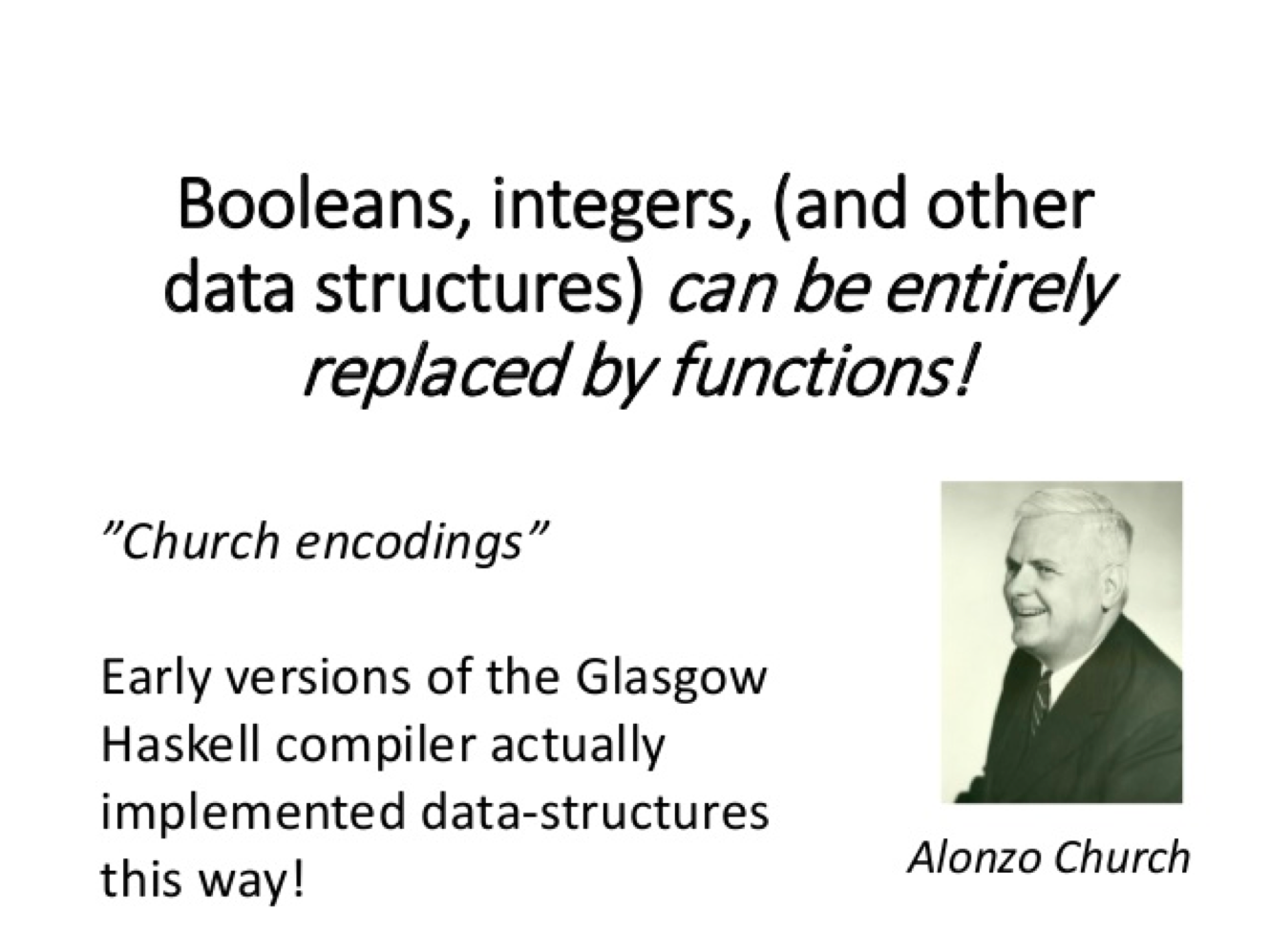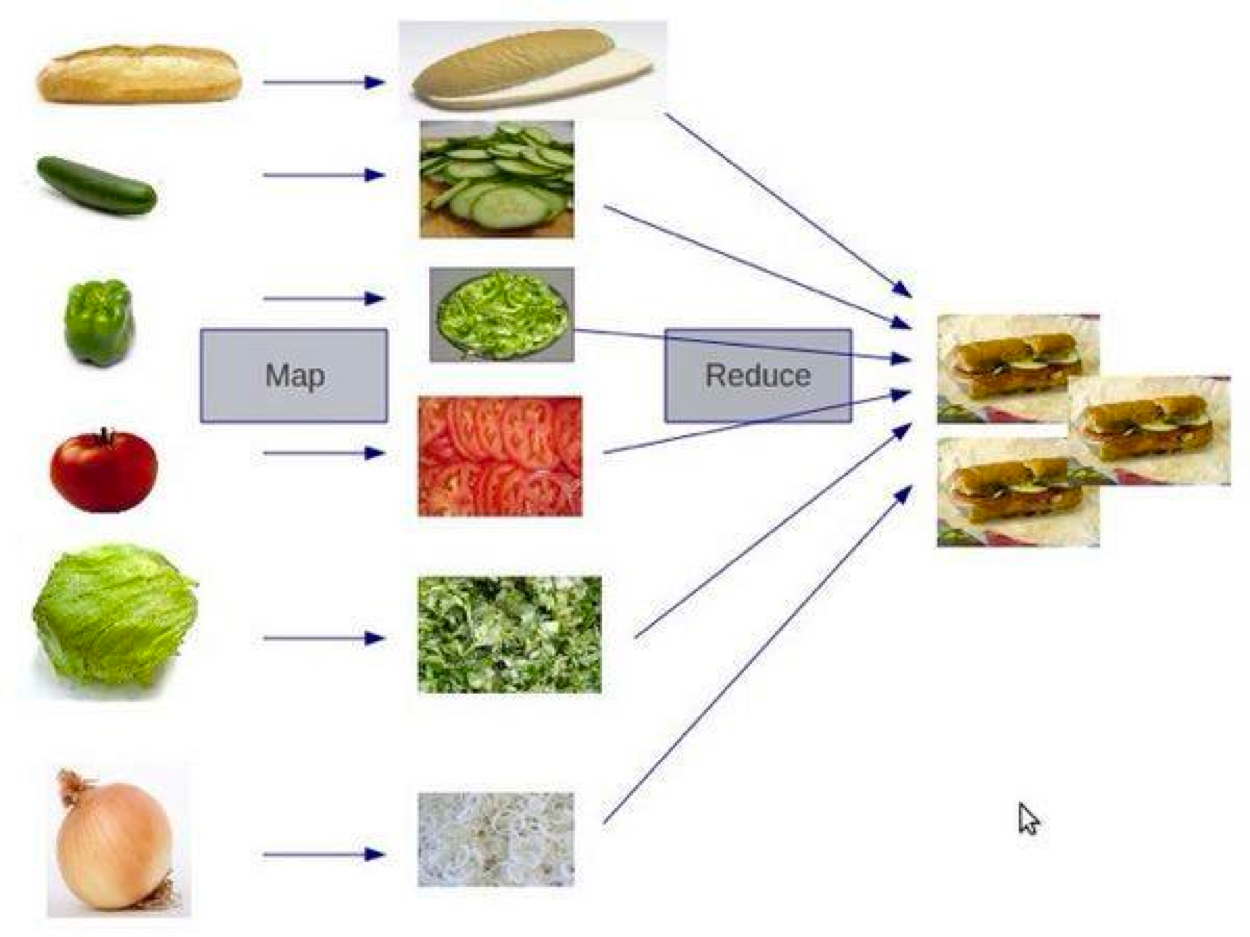033 编程范式游记（4）- 函数式编程

C++ 很大程度上解决了 C 语言中的各种问题和不便，尤其是通过类、模板、虚函数和运行时识别等解决了 C 语言的泛型编程问题。然而，如何做更为抽象的泛型呢？答案就是函数式编程（Functional Programming）。

函数式编程f(x)=5x^2+4x+3
g(x)=2f(x)+5=10x^2+8x+11
h(x)=f(x)+g(x)=15x^2+12x+14

f(x)=f(x-1)+f(x-2)

• stateless：函数不维护任何状态。函数式编程的核心精神是 stateless，简而言之就是它不能存在状态，你给我数据我处理完扔出来，里面的数据是不变的。
• immutable：输入数据是不能动的，动了输入数据就有危险，所以要返回新的数据集。

• 没有状态就没有伤害。
• 并行执行无伤害。
• Copy-Paste 重构代码无伤害。
• 函数的执行没有顺序上的问题。• 惰性求值。这需要编译器的支持。表达式不在它被绑定到变量之后就立即求值，而是在该值被取用的时候求值。也就是说，语句如 x:=expression; (把一个表达式的结果赋值给一个变量) 显式地调用这个表达式被计算并把结果放置到 x 中，但是先不管实际在 x 中的是什么，直到通过后面的表达式中到 x 的引用而有了对它的值的需求的时候，而后面表达式自身的求值也可以被延迟，最终为了生成让外界看到的某个符号而计算这个快速增长的依赖树。
• 确定性。所谓确定性，就是像在数学中那样，f(x) = y 这个函数无论在什么场景下，都会得到同样的结果，这个我们称之为函数的确定性。而不是像程序中的很多函数那样，同一个参数，却会在不同的场景下计算出不同的结果。所谓不同的场景，就是我们的函数会根据运行中的状态信息的不同而发生变化。

• 数据复制比较严重。

：有一些人可能会觉得这会对性能造成影响。其实，这个劣势并不见得会导致性能不好。因为没有状态，所以代码在并行上根本不需要锁（不需要对状态修改的锁），所以可以拼命地并发，反而可以让性能很不错。比如：Erlang 就是其中的代表。

• 容易写纯函数 F#, Ocaml, Clojure, Scala
• 纯函数需要花点精力 C#, Java, JavaScript

函数式编程用到的技术

• first class function（头等函数） ：这个技术可以让你的函数就像变量一样来使用。也就是说，你的函数可以像变量一样被创建、修改，并当成变量一样传递、返回，或是在函数中嵌套函数。
• tail recursion optimization（尾递归优化） ： 我们知道递归的害处，那就是如果递归很深的话，stack 受不了，并会导致性能大幅度下降。因此，我们使用尾递归优化技术——每次递归时都会重用 stack，这样能够提升性能。当然，这需要语言或编译器的支持。Python 就不支持。
• map & reduce ：这个技术不用多说了，函数式编程最常见的技术就是对一个集合做 Map 和 Reduce 操作。这比起过程式的语言来说，在代码上要更容易阅读。（传统过程式的语言需要使用 for/while 循环，然后在各种变量中把数据倒过来倒过去的）这个很像 C++ STL 中 foreach、find_if、count_if 等函数的玩法。
• pipeline（管道）：这个技术的意思是，将函数实例成一个一个的 action，然后将一组 action 放到一个数组或是列表中，再把数据传给这个 action list，数据就像一个 pipeline 一样顺序地被各个函数所操作，最终得到我们想要的结果。
• recursing（递归） ：递归最大的好处就简化代码，它可以把一个复杂的问题用很简单的代码描述出来。注意：递归的精髓是描述问题，而这正是函数式编程的精髓。
• currying（柯里化） ：将一个函数的多个参数分解成多个函数， 然后将函数多层封装起来，每层函数都返回一个函数去接收下一个参数，这可以简化函数的多个参数。在 C++ 中，这很像 STL 中的 bind1st 或是 bind2nd。
• higher order function（高阶函数）：所谓高阶函数就是函数当参数，把传入的函数做一个封装，然后返回这个封装函数。现象上就是函数传进传出，就像面向对象对象满天飞一样。这个技术用来做 Decorator 很不错。

// 非函数式，不是 pure funciton，有状态
int cnt;
void increment(){
cnt++;
}

// 函数式，pure function， 无状态
int increment(int cnt){
return cnt+1;
}

def inc(x):
def incx(y):
return x+y
return incx

inc2 = inc(2)
inc5 = inc(5)

print inc2(5) # 输出 7
print inc5(5) # 输出 10

• 把函数当成变量来用，关注描述问题而不是怎么实现，这样可以让代码更易读
• 因为函数返回里面的这个函数，所以函数关注的是表达式，关注的是描述这个问题，而不是怎么实现这个事情

Lisp 语言介绍

(define (plus x y)  (+ x y))
(define (times x y) (* x y))
(define (square x)  (times x x))

• 用内置的 + 函数定义了一个新的 plus 函数。
• 用内置的 * 函数定义了一个新的 times 函数。
• 用之前的 times 函数定义了一个 square 函数。

(define (f1 x) ;;; f(x) = 5 * x^2 + 10
(plus 10 (times 5 (square x))))

(define f2
(lambda (x)
(define plus
(lambda (a b) (+ a b)))
(define times
(lambda (a b) (* a b)))
(plus 10 (times 5 (times x x)))))

;;; recursion
(define factoral (lambda (x)
(if (<= x 1) 1
(* x (factoral (- x 1))))))

(newline)
(display(factoral 6))

;;; another version of recursion
(define (factoral_x n)
(define (iter product counter)
(if (< counter n)
product
(iter (* counter product) (+ counter 1))))
(iter 1 1))

(newline)
(display(factoral_x 5))

函数式编程的思维方式

传统方式的写法

from random import random

time = 5
car_positions = [1, 1, 1]

while time:
# decrease time
time -= 1

print ''
for i in range(len(car_positions)):
# move car
if random() > 0.3:
car_positions[i] += 1

# draw car
print '-' * car_positions[i]

from random import random

def move_cars():
for i, _ in enumerate(car_positions):
if random() > 0.3:
car_positions[i] += 1

def draw_car(car_position):
print '-' * car_position

def run_step_of_race():
global time
time -= 1
move_cars()

def draw():
print ''
for car_position in car_positions:
draw_car(car_position)

time = 5
car_positions = [1, 1, 1]

while time:
run_step_of_race()
draw()

函数式的写法

from random import random

def move_cars(car_positions):
return map(lambda x: x + 1 if random() > 0.3 else x,
car_positions)

def output_car(car_position):
return '-' * car_position

def run_step_of_race(state):
return {'time': state['time'] - 1,
'car_positions': move_cars(state['car_positions'])}

def draw(state):
print ''
print '\n'.join(map(output_car, state['car_positions']))

def race(state):
draw(state)
if state['time']:
race(run_step_of_race(state))

race({'time': 5,
'car_positions': [1, 1, 1]})

函数式语言的三套件

# 传统的非函数式
upname =['HAO', 'CHEN', 'COOLSHELL']
lowname =[]
for i in range(len(upname)):
lowname.append( upname[i].lower() )

# 函数式
def toUpper(item):
return item.upper()

upper_name = map(toUpper, ["hao", "chen", "coolshell"])

print upper_name
# 输出 ['HAO', 'CHEN', 'COOLSHELL']

string s="hello";
transform(s.begin(), s.end(), back_inserter(out), ::toupper);

# 计算数组中正数的平均值
num =  [2, -5, 9, 7, -2, 5, 3, 1, 0, -3, 8]
positive_num_cnt = 0
positive_num_sum = 0
for i in range(len(num)):
if num[i] > 0:
positive_num_cnt += 1
positive_num_sum += num[i]

if positive_num_cnt > 0:
average = positive_num_sum / positive_num_cnt

print average

# 计算数组中正数的平均值
positive_num = filter(lambda x: x>0, num)
average = reduce(lambda x,y: x+y, positive_num) / len( positive_num )

1. num 数组 filter 条件 x > 0 的数据。
2. 然后对 positive_num 进行 x + y 操作的 reduce，即求和。
3. ……

• 数据集、对数据的操作和返回值都放在了一起。
• 没有了循环体，就可以少了些临时用来控制程序执行逻辑的变量，也少了把数据倒来倒去的控制逻辑。
• 代码变成了在描述你要干什么，而不是怎么干函数式的 pipeline 模式

pipeline（管道）借鉴于 Unix Shell 的管道操作——把若干个命令串起来，前面命令的输出成为后面命令的输入，如此完成一个流式计算。（注：管道绝对是一个伟大的发明，它的设计哲学就是 KISS – 让每个功能就做一件事，并把这件事做到极致，软件或程序的拼装会变得更为简单和直观。这个设计理念影响非常深远，包括今天的 Web Service、云计算，以及大数据的流式计算等。）

ps auwwx | awk '{print \$2}' | sort -n | xargs echo

xargs(  echo, sort(n, awk('print \$2', ps(auwwx)))  )

pids = for_each(result, [ps_auwwx, awk_p2, sort_n, xargs_echo])

1. 找出偶数；
2. 乘以 3；
3. 转成字符串返回。

def process(num):
# filter out non-evens
if num % 2 != 0:
return
num = num * 3
num = 'The Number: %s' % num
return num

nums = [1, 2, 3, 4, 5, 6, 7, 8, 9, 10]

for num in nums:
print process(num)

# 输出：
# None
# The Number: 6
# None
# The Number: 12
# None
# The Number: 18
# None
# The Number: 24
# None
# The Number: 30

def even_filter(nums):
for num in nums:
if num % 2 == 0:
yield num
def multiply_by_three(nums):
for num in nums:
yield num * 3
def convert_to_string(nums):
for num in nums:
yield 'The Number: %s' % num

nums = [1, 2, 3, 4, 5, 6, 7, 8, 9, 10]
pipeline = convert_to_string(multiply_by_three(even_filter(nums)))
for num in pipeline:
print num
# 输出：
# The Number: 6
# The Number: 12
# The Number: 18
# The Number: 24
# The Number: 30

def even_filter(nums):
return filter(lambda x: x%2==0, nums)

def multiply_by_three(nums):
return map(lambda x: x*3, nums)

def convert_to_string(nums):
return map(lambda x: 'The Number: %s' % x,  nums)

nums = [1, 2, 3, 4, 5, 6, 7, 8, 9, 10]
pipeline = convert_to_string(
multiply_by_three(
even_filter(nums)
)
)
for num in pipeline:
print num

pipeline_func(nums, [even_filter,
multiply_by_three,
convert_to_string])

def pipeline_func(data, fns):
return reduce(lambda a, x: x(a),   fns,   data)

class Pipe(object):
def __init__(self, func):
self.func = func

def __ror__(self, other):
def generator():
for obj in other:
if obj is not None:
yield self.func(obj)
return generator()

@Pipe
def even_filter(num):
return num if num % 2 == 0 else None

@Pipe
def multiply_by_three(num):
return num*3

@Pipe
def convert_to_string(num):
return 'The Number: %s' % num

@Pipe
def echo(item):
print item
return item

def force(sqs):
for item in sqs: pass

nums = [1, 2, 3, 4, 5, 6, 7, 8, 9, 10]

force(nums | even_filter | multiply_by_three | convert_to_string | echo)